Next: Dismantlability Up: Reduction Theorems Previous: Reduction Theorems

## Removing Points

The initial work in this direction is found in the paper  by Rival, which has since been extended in various directions. We will present the best folklore version of Rival's original result in Theorem 3.2 and a modification in Theorem 3.4. Notice that chain-completeness is not needed in Theorem 3.4 and Scholium 3.5.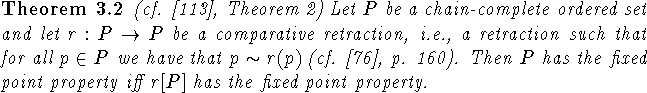Proof: The direction ``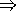" follows from part 1 of Theorem 3.1. To prove ``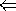" let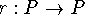be a comparative retraction and let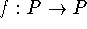be order-preserving. Then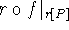has a fixed point p and since r is a comparative retraction f(p) is related to p. Thus by the Abian-Brown-Pelczar Theorem we have that f has a fixed point. \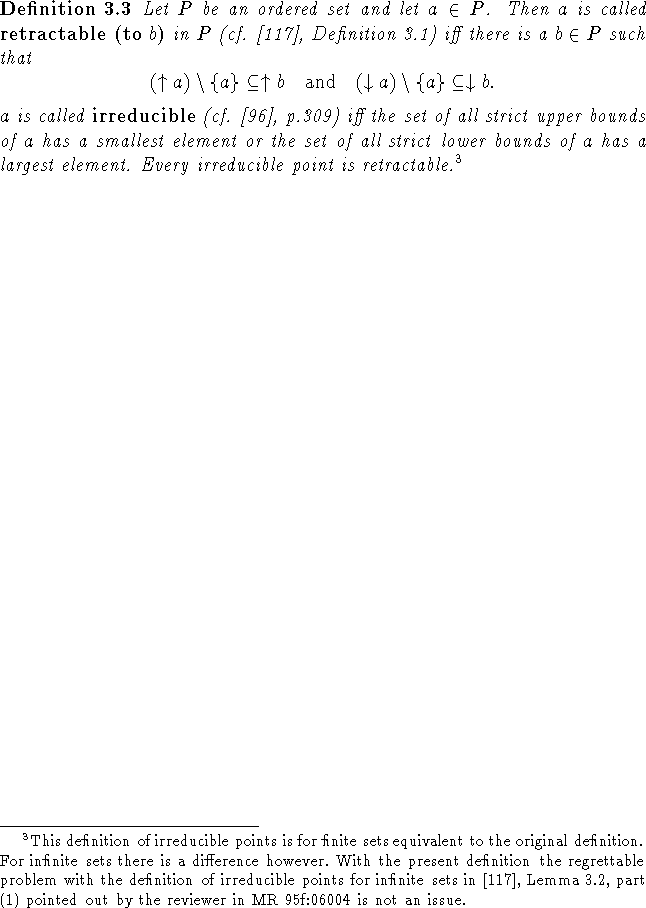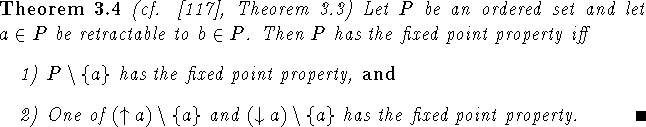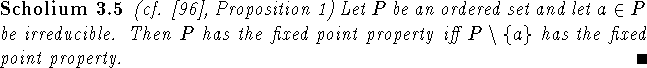Bernd.S.W.Schroder